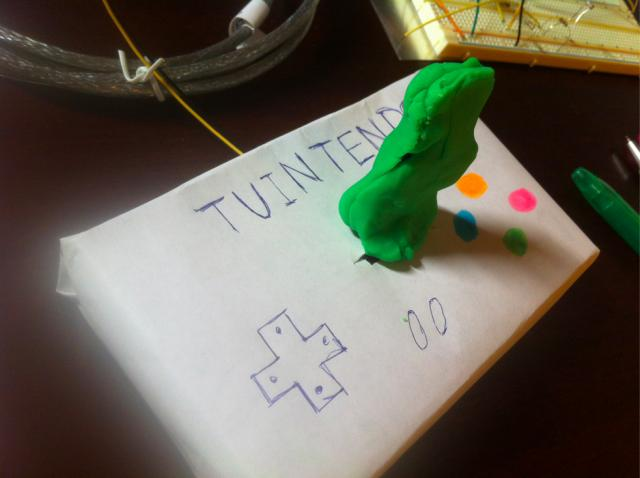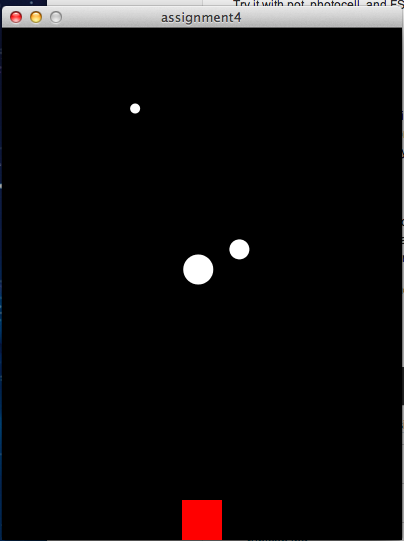# Lab4 - TUINTENDO

Submitted by jooddang on Tue, 02/26/2013 - 22:29

## Description

I reproduced a 90's console game with Arduino and Processing. A stogy controller with buttons and a joy stick and a simple design of game in a small screen evoke nostalgia of 90's games. At that time, although the games were simple and coarse, we enjoyed playing them a lot of time.

When you push the joy stick, the red rectangle arises. Your mission is to evade collision with reckless white balls!## Components

Arduino Uno

Force Sensing Resistor

10ohm resistor

a snack box

## Code

### Arduino

#include <SoftwareSerial.h>

/*
* Resistive Sensor Input
* Takes the input from a resistive sensor, e.g., FSR or photocell
* Dims the LED accordingly, and sends the value (0-255) to the serial port
*/

int photoPin = 0;
int forcePin = 1;  // select the input pin for the sensor
int ledPin = 11;    // select the output pin for the LED
int photoval = 0;
int forceval = 0;        // variable to store the value coming from the sensor
//SoftwareSerial forceSerial(0,1);

void setup() {
Serial.begin(9600);
//  forceSerial.begin(14400);
}

void loop() {
//  forceval = analogRead(forcePin); // read the value from the sensor, 0-1023
//  analogWrite(ledPin, val/4);  // analogWrite (dimming the LED) can be between 0-255
Serial.println(photoval/2);       // writing the value to the PC via serial connection
//  forceSerial.println(forceval/4);
delay(50);                   // rest a little...
}

### Processing

import processing.serial.*;
// Change this to the portname your Arduino board
//String portname = "/dev/tty.usbserial-A4001nLJ"; // or "COM5"
String portname = "/dev/tty.usbmodemfa131"; // or "COM5"
Serial port;
String buf="";
int cr = 13;  // ASCII return   == 13
int lf = 10;  // ASCII linefeed == 10

MovingCircle myCircle = new MovingCircle(50,50,10);
MovingCircle myCircle2 = new MovingCircle(150,150,20);
MovingCircle myCircle3 = new MovingCircle(250,250,30);

int force = 0;
int c = 0;
int h = 0;

void setup() {
size(400,512);
frameRate(50);
smooth();
background(40,40,40);
noStroke();
port = new Serial(this, portname, 9600);

fill (255,0,0);
h = height - 40;
rect (width / 2 - 20, h, 40, 40);
}

void draw() {
background(0);

// update the position of the circles
myCircle.update();
myCircle2.update();
myCircle3.update();

// check for collisions with the walls
myCircle.checkCollisions();
myCircle2.checkCollisions();
myCircle3.checkCollisions();

// and draw each one
myCircle.drawCircle();
myCircle2.drawCircle();
myCircle3.drawCircle();

if (c != lf && c != cr)
force = c;
if (force <= 48) {
h = h + 6;
if (h > height - 40)
h = height - 40;
}
else {
h = h - 2;
if (h < 0)
h = 0;
}
fill (255,0,0);
rect (width / 2 - 20, h, 40, 40);
}

void keyPressed() {
if(key == ' ') {
background(40,40,40);  // erase screen
}
else {
int x = int(random(0,width));
int y = int(random(0,height));
drawball(x,y, 50);
}
}

// draw balls
void drawball(int x, int y, int r) {
for (int i=0; i<100; i++ ) {
fill(255-i,i,240);
ellipse(x,y,r,r);
}

}

// called whenever serial data arrives
void serialEvent(Serial p) {
if (c != lf && c != cr && c != 48)
println("val=" + c);

/*
if (c != lf && c != cr) {
buf += char(c);
}
if (c == lf) {
int val = int(buf);
println("val="+val);
int x = int(random(0,width));
int y = int(random(0,height));
drawball(x,y,val);
buf = "";
background(40,40,40);  // erase screen
}
*/
}

// start with the name of the class (or type of object)

class MovingCircle {

// any variable declared here will be properties of
// objects of this type
float x;
float y;
float xSpeed;
float ySpeed;
float circleSize;

// this special function declaration has the same name
// as the class (MovingCircle) and it has no return type. This
// is known as the constructor and it's run when an
// object of this type is created.

MovingCircle(float xpos, float ypos, float csize) {
x = xpos;
y = ypos;
circleSize = csize;

xSpeed = random(-7, 7);
ySpeed = random(-7, 7);

}

// update adds the speed to the position, making
// our circle move around.
void update() {
x += xSpeed;
y += ySpeed;
}

// this function checks to see if our circle has gone off
// the edge of the screen, and if so reverses the speed

void checkCollisions() {
float r = circleSize/2;

if ( (x<r) || (x>width-r)){
xSpeed = -xSpeed;
}

if( (y<r) || (y>height-r)) {
ySpeed = -ySpeed;
}
}

void drawCircle() {
fill(255);
ellipse(x, y, circleSize, circleSize);

}
}